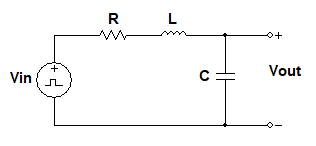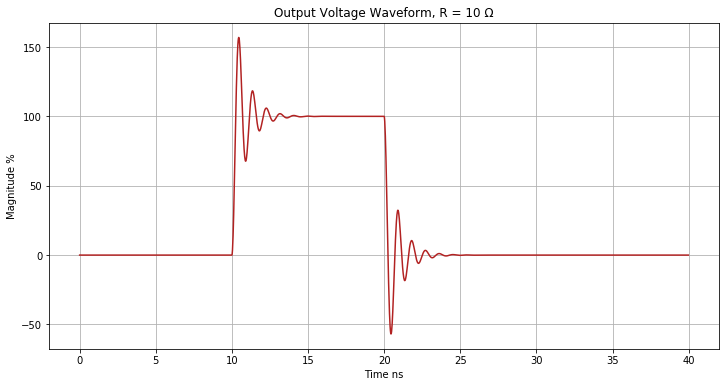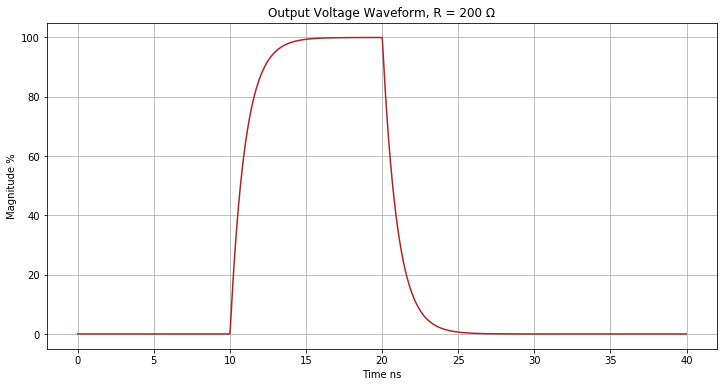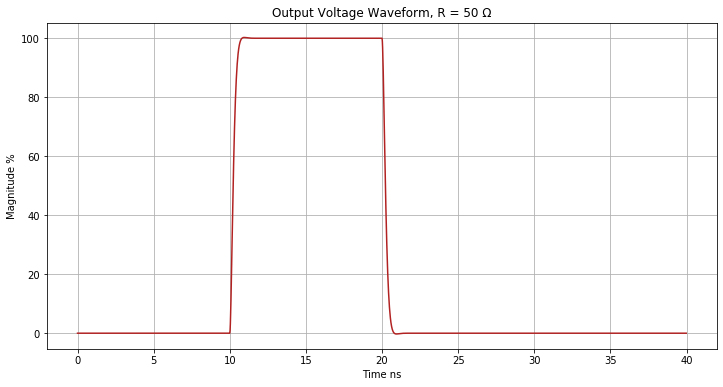## Sunday, May 30, 2021

### Pulse Response Examiner - a Python Jupyter Notebook

This notebook simulates the pulse response of a basic RLC circuit.You can use this notebook to help you choose a resistor R that you solder in series at your pulse-generating source, to minimize ringing overshoot (due to inductance) while not rounding the pulse edges too much (due to capacitance). In the last plot below, we find a good resistance value for R.

### Define the function that performs an inverse Laplace transform¶

# PULSE_RESPONSE_EXAMINER PROGRAM
# Version 1.0.1, 02-Jul-2021
# Contact: xejgyczlionautomeasuretigercom replace lion with
# an at sign and tiger with a dot.
#
# you may not use this file except in compliance with the License.
# You may obtain a copy of the License at
#
#
# Unless required by applicable law or agreed to in writing, software
# WITHOUT WARRANTIES OR CONDITIONS OF ANY KIND, either express or implied.
# See the License for the specific language governing permissions and

import math, cmath
import numpy as np
from numpy.fft import fft
import matplotlib.pyplot as plt

# FUNCTION inv_laplace(): employs the 'fourier method' for inversion of the Laplace
# transform. The algorithm here is an adaptation by Joe Czapski of a Mathcad
# worksheet by Sven Lindhardt. It's a practical approximation of inverse Laplace for
# systems close to stability, whether a little overstable or understable, when the
# rightmost pole is not greater than zero. NumPy's complex FFT is used in this
# application to generate a fast inverse Laplace transform.
def inv_laplace(n, R, L, C, t1, t2, tspan):
# Set error correction factor to reduce artifacts when both L and C are tiny.
efactor = 2.5
# Calculate the complex Vout(s) using the transfer function of the circuit
# and the Laplace transform of the input waveform.
sigma = efactor / tspan
domega = math.pi / tspan   # angular frequency sample interval
Vouts = np.zeros(n+1, dtype=np.cdouble)
for i in range(0, n+1):
omega = i * domega
s = sigma - omega*1.0j
sL = s * L
sCinv = 1.0 / (s * C)
# Calculate H(s) for the desired circuit.
# For an RLC circuit H(s) = (1/sC) / (R + sL + 1/sC)
Hs = sCinv / (R + sL + sCinv)
# Calculate Vin(s) for the input waveform.
# For an ideal pulse Vin(s) = 100 * (e^-t1s - e^-t2s) / s
Vins = 100.0 * (cmath.exp(-t1*s) - cmath.exp(-t2*s)) / s
# Calculate Vout(s) = H(s) * Vin(s)
Vouts[i] = Hs * Vins
# Perform the complex fourier transform. Before calling fft(),
# construct a symmetric version of complex array Vouts.
Vouts[n] = Vouts[n].real + 0.0j
Vouts_symmetric = np.concatenate([Vouts, (Vouts[1:n])[::-1]])
Vout_unscaled = fft(Vouts_symmetric)
# Take the real part of Vout_unscaled, first half of the symmetric array,
# and scale for inverse Laplace using time span, sigma, and exp function.
# The sample interval in seconds = tspan / n
sigdx = efactor / n
Vout = np.zeros(n)
for i in range(0, n):
Vout[i] = math.exp(i*sigdx) * Vout_unscaled[i].real / tspan
return Vout


### Set the circuit component values, pulse width, and time scale, and generate the plot¶

You can use this handy inductance calculator for your cabling or traces situation. In the formulas on that webpage, use µr = 1 if the intervening material is air or a dielectric insulator.

R = 10.0   # ohms
L = 4.0e-9   # henries
t1 = 1.0e-8   # pulse rising edge seconds from t0
t2 = 2.0e-8   # pulse falling edge seconds from t0
# Set tspan which is the time span of the analysis in seconds from t0.
# Artifacts can arise when Vout doesn't settle to zero before the end of the
# analysis time. You may need to adjust tspan to increase the analysis time
# following the end of the pulse to allow settling to zero.
tspan = 4.0e-8
# Set n which is the number of points to use for the analysis. n must be a power of 2.
# Set n=2048 for most cases. Set n=8192 to reduce artifacts when both L and C are tiny.
n = 2048

ns_per_s = 1.0e9   # time display unit correction
xdata = np.linspace(0.0, tspan * ns_per_s * (n-1)/n, n)
ydata = inv_laplace(n, R, L, C, t1, t2, tspan)

plt.rcParams['figure.figsize'] = [12, 6]
plt.title('Output Voltage Waveform, R = %d Ω' % (R))
plt.xlabel('Time ns')
plt.ylabel('Magnitude %')
plt.grid(True)
plt.plot(xdata, ydata, color='firebrick')
plt.show()### Try different values of R, regenerating the plot for each value¶

R = 200.0   # ohms
ydata = inv_laplace(n, R, L, C, t1, t2, tspan)
plt.title('Output Voltage Waveform, R = %d Ω' % (R))
plt.xlabel('Time ns')
plt.ylabel('Magnitude %')
plt.grid(True)
plt.plot(xdata, ydata, color='firebrick')
plt.show()R = 50.0   # ohms
ydata = inv_laplace(n, R, L, C, t1, t2, tspan)
plt.title('Output Voltage Waveform, R = %d Ω' % (R))
plt.xlabel('Time ns')
plt.ylabel('Magnitude %')
plt.grid(True)
plt.plot(xdata, ydata, color='firebrick')
plt.show()